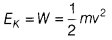# Show that the kinetic energy of mass 'm' moving with velocity 'v' is

Show that the kinetic energy of mass ‘m’ moving with velocity ‘v’ is given by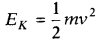Let m = mass of the body,
u = initial velocity of the body,
F = force applied on the body,
a = acceleration produced in the body in the direction of force,
v = final velocity of the body
and s = distance covered by the body.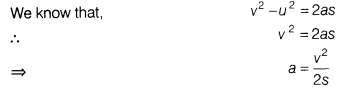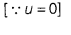As, force and displacement are in the same direction, the work done on the body is positive.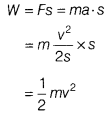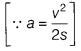Thus, the work done appears as the kinetic energy of the body.